Two-Sided Fixed-Sample Tests in Clinical Trials

A two-sided test is a test of a hypothesis with a two-sided alternative. Two-sided tests include simple symmetric tests and more complicated asymmetric tests that might have distinct lower and upper alternative references.

### Symmetric Two-Sided Tests for Equality

For a symmetric two-sided test with the null hypothesis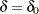against the alternative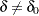, an equivalent null hypothesis is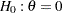with a two-sided alternative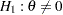, where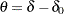. A fixed-sample test rejects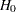if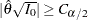, where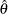is a sample estimate of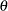and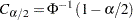is the critical value.

A common two-sided test is the test for the response difference between a treatment group and a control group. The null and alternative hypotheses areand, respectively, whereis the response difference between the two groups. If a greater value indicates a beneficial effect, then there are three possible results:

• The test rejects the hypothesisof equality and indicates that the treatment is significantly better if the standardized statistic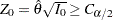.

• The test rejects the hypothesisand indicates the treatment is significantly worse if the standardized statistic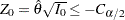.

• The test indicates no significant difference between the two responses if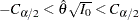.

The-value of the test is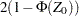if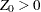and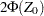if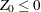. The hypothesisis rejected if the-value of the test is less than—that is, if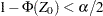or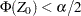. A symmetric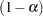confidence interval forhas lower and upper limits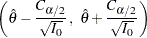which is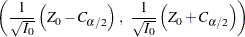The hypothesisis rejected if the confidence interval for the parameterdoes not contain zero. That is, the lower limit is greater than zero or the upper limit is less than zero.

With an alternative reference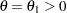, a Type II error probability is defined as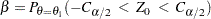which is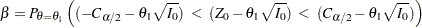Thus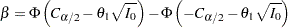The resulting power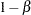is the probability of correctly rejecting the null hypothesis, which includes the probability for the lower alternative and the probability for the upper alternative. The SEQDESIGN procedure uses only the probability of correctly rejecting the null hypothesis for the correct alternative in the power computation.

Thus, under the upper alternative hypothesis, the power in the SEQDESIGN procedure is computed as the probability of rejecting the null hypothesis for the upper alternative,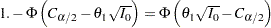, and a very small probability of rejecting the null hypothesis for the lower alternative,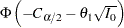, is ignored. This power computation is more rational than the power based on the probability of correctly rejecting the null hypothesis (Whitehead 1997, p. 75).

That is,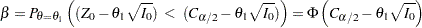Then with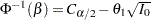,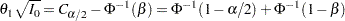The drift parameter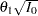can be derived for specifiedand, and the maximum information is given by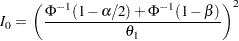If the maximum information is available, then the required sample size can be derived. For example, in a one-sample test for mean, if the standard deviation
is known, the sample sizerequired for the test is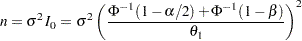On the other hand, if the alternative reference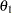, standard deviation, and sample sizeare all known, thencan be derived with a givenand, similarly,can be derived with a given.

### Generalized Two-Sided Tests for Equality

For a generalized two-sided test with the null hypothesisagainst the alternative, an equivalent null hypothesis is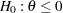with a two-sided alternative, where. A fixed-sample test rejectsif the standardized statistic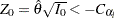or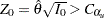, where the critical values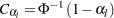and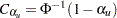.

With the lower alternative reference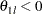, a lower Type II error probability is defined as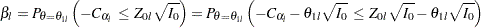This implies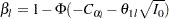and the power is the probability of correctly rejecting the null hypothesis for the lower alternative,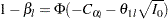The lower drift parameter is derived asThen, with specified
and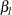, if the maximum information is known, the lower alternative reference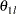can be derived. If the maximum information is unknown, then with the specified lower alternative reference, the maximum information required is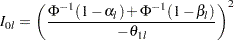Similarly, the upper drift parameter is derived as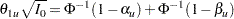For a given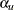,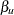, and the upper alternative reference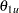, the maximum information required is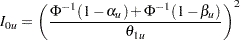Thus, the maximum information required for the design is given by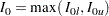Note that with the maximum information level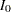, if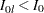, then the derived power from the lower alternative is larger than the specified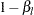. Similarly, if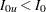, then the derived power from the upper alternative is larger than the specified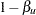.

If maximum information is available, the required sample size can be derived. For example, in a one-sample test for mean, if the standard deviationis known, the sample sizerequired for the test is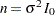.

On the other hand, if the alternative references, Type I error probabilities
and, standard deviation, and sample sizeare all specified, then the Type II error probabilitiesandand the corresponding powers can be derived.